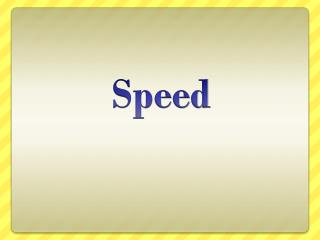DownloadDownload PresentationSpeed

# Speed

Download Presentation## Speed

- - - - - - - - - - - - - - - - - - - - - - - - - - - E N D - - - - - - - - - - - - - - - - - - - - - - - - - - -
##### Presentation Transcript

1. Speed

2. Speed • Speed: the rate at which an object moves • Anything that is changing its position has speed • Example: ocean currents, the moon, a car, a person walking

3. Speed • Formula: speed = distancetime • Constant Speed: speed that does not change • Average Speed: dividing the total distance by the total time

4. Measuring Motion Sample problem: At what speed did a plane fly if it traveled 1760 meters in 8 seconds? Step 1 – Write the formula. Speed = Distance/Time Step 2 – Substitute given numbers and units. Speed = 1760 meters/8 seconds Step 3 – Solve for the unknown. Speed = 220 meters/second (m/sec)

5. Measuring Motion Practice Problems: • A car travels 240 kilometers in 3 hours. What is the speed of the car during that time? • The speed of a cruise ship is 50 km/hr. How far will the ship travel in 14 hours?

6. A car travels 240 kilometers in 3 hours. What is the speed of the car during that time? Step 1: Speed = Distance/Time Step 2: Speed = 240 km/3hrs Step 3: Speed = 80 km/hr Answer: The speed of the car is 80 km/hr.

7. The speed of a cruise ship is 50 km/hr. How far will the ship travel in 14 hours? Step 1: Speed = Distance/Time Step 2: Speed = 50km/hr Step 3: 50 km x 14 hrs = 700km/14 hrs Answer: The ship will travel 700 km in 14 hrs.

8. Measuring Motion • The speed of a moving object is not always constant. • For example, a cyclist takes a long bike ride. She begins slowly for the first two hours and picks up speed for the third hour. After three hours, she takes a break before finishing the final two hours of her ride.

9. Measuring Motion • Clearly, her speed changes many times throughout her journey. • Dividing the total distance by the total time does not tell you her speed for every point of her journey. • Instead it gives you her average speed. • In fact, the formula you just learned for calculating speed – distance/time – always gives you the average speed.